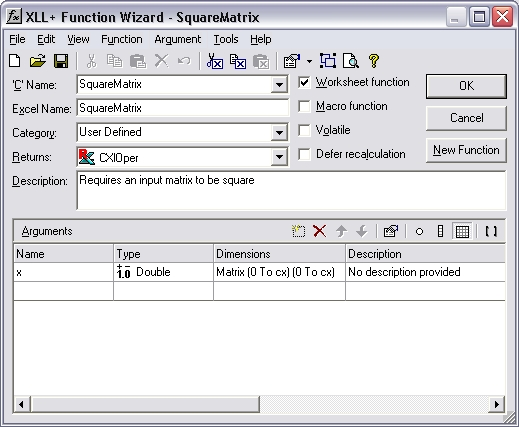HOW TO: How can I make sure that a matrix input is square?

Reference: Q0037

• XLL+ for Visual Studio 2005 - 5.0
• XLL+ for Visual Studio .NET - 4.2, 4.3.1, 5.0
• XLL+ for Visual Studio 6 - 3, 4.1, 4.2, 4.3.1, 5.0

HOW TO: How can I make sure that a matrix input is square?

Question

My add-in function expects a matrix input to have same number of rows as it has columns. Can I use the Function Wizard to specify this constraint?

You can modify your arguments to be bounded input arrays. The XLL+ Function Wizard has a tab which lets you set the bounds of an array, so that they are tied to a named variable (or to a constant). The code which is generated will automatically check that all matrix and vector inputs satisfy the constraints you apply.

By using the same named variable for the height of the input and also for its width, you can ensure that height of the input is equal to its width.

If the input is not square, then an error value is returned, e.g. if x has 3 rows and 4 columns:

#Error: expected 3 columns in x

Example

Problem: constrain the height of a matrix to equal its width.

Steps

1. Create a new function, SquareMatrix(), using the XLL+ Function Wizard. It takes one argument x, which is defined as a matrix of doubles.2. Select the Dimensions column of the row containing the definition of x, and click on the ... button. (Or use the short-cut Ctrl+E.) This will display the Edit Argument dialog.

Select the Array tab.

In the Rows box, check the Bounded check-box and set the upper bound (UBound) to be cx, as shown below.

In the Columns box, check the Bounded check-box and set the upper bound (UBound) also to be cx, as shown below.3. Press OK to close the dialog.
4. Inspect the changes to the Function Wizard. The Dimensions column now contains the new definition of x: Matrix (0 to cx)(0 to cx).5. Save the function.

Code

The code generated is shown below:

// Function:    SquareMatrix
// Purpose:     Requires an input matrix to be square

//{{XLP_SRC(SquareMatrix)
// NOTE - the FunctionWizard will add and remove mapping code here.
//    DO NOT EDIT what you see in these blocks of generated code!
IMPLEMENT_XLLFN2(SquareMatrix, "RP", "SquareMatrix", "x",
"User Defined", "Requires an input matrix to be square",
"No description provided\000", "B0(0,cx)0(0,cx)x No descripti"
"on provided\0appscope=1\0", 1)

extern "C" __declspec( dllexport )
LPXLOPER SquareMatrix(const COper* x)
{
XLL_FIX_STATE;
CXlOper xloResult;
BOOL bOk = TRUE;
long cx = -1;
MTX_PTRS<double> matx;
bOk = bOk && x->ReadMatrix(matx, "x", xloResult, &cx, &cx, XLA_ARRAY_FLAGS_NUMERIC_STD, 0, 0);
if (!bOk)
return xloResult.Ret();
//}}XLP_SRC

// TODO - Set the value of xloResult
return xloResult.Ret();
}

Explanation

The interesting code is shown in bold above.

1. The bounds variable cx, which we entered (twice) as UBound in the Edit Argument dialog, is declared, and is initialized to -1. This value represents "not yet known".
2. A reference to cx is passed to COper::ReadMatrix as the value for plUBound1 and also for plUBound2.
3. When ReadMatrix inspects the number of rows in x, the bound value cx contains -1 (meaning "not yet known"), and ReadMatrix simply sets its value to the height of x.
4. Next, ReadMatrix inspects the number of columns in x and compares it to cx (which now contains the number of rows in x). Since cx contains a non-negative integer, it is treated as a fixed size constraint. If x does not contain cx columns then an error is returned.

Result

If, for example, x contains 3 rows and 4 columns, then the following error value is returned

#Error: expected 3 columns in x

and the function does not continue.